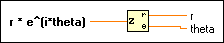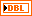# Complex To Polar Function

## LabVIEW 2018 Help

Edition Date: March 2018
Part Number: 371361R-01
View Product Info

### DOWNLOAD (Windows Only)LabVIEW 2016 HelpLabVIEW 2017 HelpLabVIEW 2018 HelpLabVIEW 2019 HelpLabVIEW 2020 Help

Owning Palette: Complex Functions

Requires: Base Development System

Breaks a complex number into its polar components.

The connector pane displays the default data types for this polymorphic function.r * e^(i*theta) can be a complex number, a cluster of complex numbers, an array of complex numbers, an array of clusters of complex numbers, and so on.r is of the same data type structure as r * e^(i*theta), with scalar representation instead of complex.theta is of the same data type structure as r * e^(i*theta), with scalar representation instead of complex. theta is in radians.

## Complex To Polar Details

Given z in rectangular form z = a + bi, this function converts the polar components of z = r * e^(i*theta) according to the following equations:

r = |z| = sqrt(a² + b²)

theta = arg(z) = arctan2(b,a)

When you wire matrix data as an input to this function, a VI that includes subVIs that work with the matrix data type replaces the function. The resulting VI has the same icon but contains a matrix-specific algorithm. The node remains a VI if you disconnect the matrix from the input(s). Wire other data types as inputs to restore the original function. If you wire a data type to a function and that data type causes a basic math operation to fail, the function returns an empty matrix or NaN.

Not Helpful